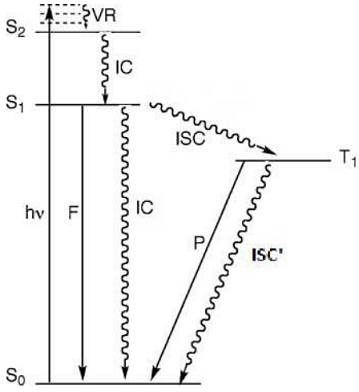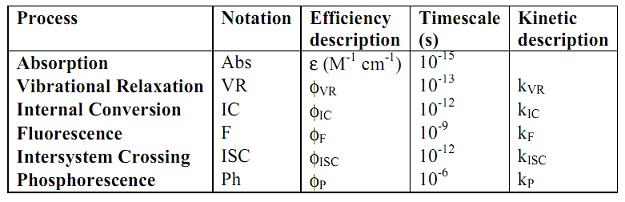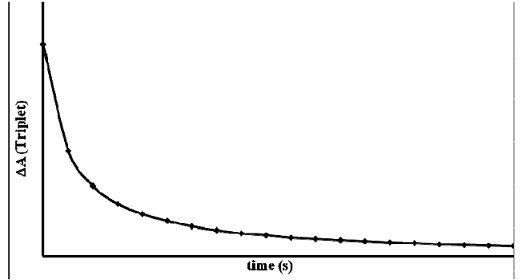#### Selection rules-photophysical parameters, Chemistry tutorial

INTRODUCTION

We have seemed at the way photons are absorbed. Photons of UV and visible light cause electrons to promote between orbitals. In this Unit, we shall look at the way this photon absorption is analysed via spectroscopists. When a photon is absorbed or produced, we must preserve the total angular momentum in the overall procedure. So we must create via looking at several of the 'rules' that permit for intense UV-visible bands (caused via electronic motion). Selection rules are a set of restrictions governing the likelihood that a physical system will transform from one state to another or will be unable to make these a transition. Selection rules, accordingly, might identify "allowed transitions," those that have an elevated probability of occurring, or "forbidden transitions," those that have minimal or no probability of occurring. It is advisable to utilize a high-power lamp when performing a photochemical reaction since it generates more photons than a low-power lamp. Its flux is greater. When we looked at the laws of photochemistry, we saw how the 2nd law stated the thought that when a species absorbs radiation, one particle is excited for each quantum of radiation absorbed. This (hopefully) obvious truth now requires to be investigated further. The 'quantum yield' Φ is a helpful idea for quantifying the number of molecules of reactant consumed per photon of light. It might be described mathematically via

Selection rules

A selection rule is a quantum mechanical rule that explains the kinds of quantum mechanical evolutions that are permitted. Transitions not permitted through selection rules are said to be "forbidden," although in practice, such transitions are usually forbidden 'to first order' only, which means they may occur in practice but with low probability. Light absorption is a resonant process; hence the most fundamental condition for absorption is that the energy of the incoming photon must match the dissimilarity in energy between the 2 energy states included in the transition (?E = hν). In calculation to this energy matching, the probability of an electronic transition is predicted using a set of selection rules, which might be summarized as follows:

Spin selection rule (ΔS = 0)

Transitions involving spin changes (S→T, or T→S) are strongly forbidden. This rule can be relaxed in the presence of heavy atoms and paramagnetic species. The fact that spin-forbidden transitions can be observed at all is as a result of the phenomenon of spin-orbit coupling, which is the interaction of the electron's spin magnetic moment with its orbital magnetic moment. This interaction introduces a new term into the Hamiltonian operator which operates on both spin and space variables. In the presence of this new term, the zero-order wave functions of the system, which were hitherto pure singlets and triplets, are combined to form new wave functions of mixed multiplicity. S→T and T→S transitions therefore become somewhat probable because the singlet state is no longer pure singlet but has some triplet character mixed with it. The triplet state also has some singlet character.

LaPorte selection rule (g↔u; Δl = ±1)

Whether spin-permitted or spin-forbidden, several evolutions (for example d-d) are usually extremely weak. This could be due to the LaPorte rule that forbids evolutions including no change of parity (p↔p, d↔d, f↔f). If the sign of the wave function varies on reflection through a centre of smmetry, then the symmetry is termed to as ungerade signified u; if it is unchanged, it is said gerade denoted g. Then according to this rule, g→u and u→g transitions are permitted, while u→u and g→g transitions are forbidden. The corollary of the LaPorte rule is that d↔p and s↔p transitions are permitted, while d↔d, and s↔d transitions are forbidden. The selection rule which states that transitions which cause a huge change in linear of angular momentum of the molecule are forbidden is as well consistent by the LaPorte rule. Though, the LaPorte rule doesn't harshly relate in the presence of minor static distortions or non-totally symmetric vibrational motions that eliminate the exact centrosymmetry. This could be accountable for the examined intensities of the d→d transitions in octahedral complexes.

Franck-Condon Selection Rule

The conventional Franck-Condon principle needs that, since an electronic evolution is "instantaneous", the nuclear coordinates and momenta do not change. As a consequence of this transition, electron density is quickly built up in new regions of the molecule and eliminated from others. The change in electron density causes a new force field on the stationary nuclei, which causes the molecules to vibrate. The most probable evolution is from the ground state to the vibrational state lying perpendicularly above it. In terms of the quantum mechanics, transitions are most probable when the wave function of the upper vibrational state most intimately resembles the ground state vibrational wave function.

Orbital Overlap Selection Rule

Orbitals included in an electronic transition require overlapping. For instance, the π→π* transition in carbonyls is extremely probable since the orbitals included do overlap. This isn't the case for the n→π* transition that is forbidden.

The Quantum Yield

The quantum yield (Φ) of a radiation-induced procedure is the number of times a specific event occurs per photon absorbed by the system. The 'event' is classically a type of photophysical or photochemical procedure. By definition, quantum yield is the number of described events that take place per photon absorbed via the system. The quantum yield (φ) symbolizes and quantifies the competence of a photophysical or photochemical procedure.

Absorbed photons of number events of number = φ

This description emanates from the 1st and 2nd laws of photochemistry, which suggested that for any photochemical procedure to take place, there must be absorption of photons, and that one photon activates only one molecule. Many features, intrinsic and extrinsic to the system, can affect the efficiency of a photochemical procedure. Quantum yield is a compute of the competence of these processes after light absorption. The efficiencies of all photochemical and photophysical processes are quantified via the quantum yield. Its value can lie anywhere in the range 10-6 to 106. A value of 10-6 implies that the photon absorption procedure is extremely inefficient through only one molecule absorbed per million photons.

In other words, the energetic requirements for reaction aren't being met. On the other hand, a quantum yield Φ of greater than unity can't be attained during a straightforward photochemical reaction because the 2nd law of photochemistry clearly tells that one photon is consumed per species excited. Actually, values of Φ > 1 indicate, which a secondary reaction(s) has happened. A value of Φ > 2 implies that the product of the photochemical  reaction is devoured via another molecule of reactant, for example during a chain reaction, through one photon generating a easy molecule of, say, excited chlorine, which cleaves in the excited state to produce 2 radicals. Each radical then reacts in propagation reactions until the reaction mixture is drained of reactant.

To assist illuminate the condition, we usually describe 2 kinds of quantum yield: primary and secondary. The magnitude of the primary quantum yield terms solely to the photochemical formation of a product so, from the 2nd law of Photochemistry, the value of Φprimary can't be greater than unity. The so-called secondary quantum yield terms to the total number of product molecules shaped via secondary (chemical) reactions; its value isn't limited. The primary quantum yield should always be cited mutually through the photon pathway occurring: it is general for numerous possible pathways to coexist through each characterized via a divide value of Φ. As a natural consequence of the 2nd law of photochemistry, the sum of the primary quantum yields can't be greater than unity.

The quantum yield of a photophysical procedure is straight related to the 'allowedness' of the procedure. For instance, phosphorescence (T→S transition) is a spin-forbidden process; therefore its quantum yield is supposed to be small. The quantum yields for several photophysical and photochemical procedures are described therefore:

Fluorescence quantum yield: Φf   = no of fluorescing molecules/ no of photons absorbed

Internal conversion: φIC   = no of molecules undergoing internal conversion / no of photons absorbed

Intersystem crossing: φ ISC = no of molecule undergoing intersystem crossing / no of photons absorbed

Phosphorescence: φP = no of phosphorescing molecules / no of photons absorbed

Photoreaction:  φR = no of molecules undergoing photoreact / no of photons absorbed

Calculating the Number of Photons Absorbed

Photons are the particles of light. Properties of photons:

• Photons always shift through the speed of light.
• Photons are electrically neutral.

Two pieces of information are required to find out the number of photons absorbed via an instance:

1.  The power (or energy) of the incident light Light emanating from a source (laser, flash light, incandescent light bulb, and so on is characterized via its power output (for example 80 W, 80 Js-1).

The relationship between power (J s-1 or W) is energy of an incident radiation is:

Eincident (J) = Powerincident (J s-1) x time (s).

2.  The quantity of energy per emitted photon (energy of 1 photon)

Photons have no mass, but they have energy E = hν = hc/λ. Here h = 6.626 x10-34Js is a universal constant called Planck's constant.  The energy of each photon is inversely proportional to the wavelength of the associated electromagnetic wave.  The shorter the wavelength, the more energetic is the photon, the longer the wavelength, the less energetic is the photon.

Ephoton = hvphoton = hc/   λphoton

Knowing such 2 quantities, then the number of photons incident photons (Nincident) is specified as:

Nincident = Eincident / Ephoton

The number of photons absorbed (Nabsorbed) is specified as

Nabsorbed = α.Nincident

Where α is the fraction of incident photons absorbed for instance, the competence of absorption. α is sometimes specified as  %-absorption.

In the field of photochemistry, two significant excited states are recognized: the singlet and the triplet excited states. The lifetime of such states is designated τF and τT correspondingly. Though, there exists a term said the radiative lifetime, allocated as τ0. The photophysical procedures that deactivated the 1st excited singlet state are:

S0 + hν   →    S1                               Rate = Ia                       Absorption

S1          →    S0 + hνF                      Rate = kF[S1]                Fluorescence

S1       --→    S0 + heat                    Rate = kIC[S1]               Internal conversion

S1       --→    T1                               Rate =  kISC[S1]             Intersystem crossing

The radiative lifetime, τ0, is a compute of the probability of emission that is related to the probability of absorption. The radiative lifetime is symbolized via:

τ0 = 1/kF

By definition, τ0 is the lifetime of an excited molecule in the absence of radiationless evolutions. The radiative lifetime isn't the definite lifetime of the excited state; it is the lifetime if fluorescence was the only procedure deactivating the excited state, and it is the reciprocal of the 1st order rate steady of fluorescence. Subsequent excitation, the molecule will as well relax via internal conversion and intersystem crossing, in addition to fluorescence; so that the rate of decay of the singlet stimulated state is the sum of such three decay procedures.

-d [S]/dt=(kf + k IC  + k ISC [S1]Figure X: Jablonski diagram showing processes at the S1 and T1 states

The lifetime of the excited state (S1) is the reciprocal of the 1st order rate steady for decay.

τ= 1/ kF  + k ISC + kIC

However, τ0 = 1/kF

There

The triplet state (T1) formed can undergo further relaxation procedures:

 T1 → S0 + hνP         Rate = kP[T1]                         Phosphorescence (radiative) T1 → S0                   Rate = k′ISC[T1]                     Intersystem crossing (non-radiative)

Where kP is the rate steady for phosphorescence; and k′ISC is the rate constant for intersystem crossing from T1 state to S0.

Typically, the rate of triplet formation is much faster than the rate of triplet decay, for example, kISC > (kP + k′ISC).

As in the case of the singlet state,

-d[T1]/dt =  (kp + k'ISC) [T1]

And the phosphorescence (triplet) lifetime is specified via:

τI = 1/ kp + k'ISC

In all cases, the excited triplet state is longer lived than the singlet counterpart since of the decay (radiative or non-radiative) of the T1 to the S0 state is forbidden. Table X provides a description of the general photophysical procedures.

Table X: Description of the regular photophysical propertiesExponential Decay of the Excited State

The lifetime (τ) of a chemical species, which decays in a first-order process, is the time needed for a concentration of this species to decrease to 1/e of its original value. Statistically, τ represents the mean life expectancy of an excited species. In a reacting system in which the decrease in concentration of a particular chemical species is governed by a first-order rate law, τ is equal to the reciprocal of the sum of the (pseudo) unimolecular rate constants of all processes, which cause the decay.

For any molecular entity which decays in a first order kinetics,

Rate of decay = -dA/dt =kd [A]

kd is the 1st order decay constant. Rearranging this equation, we have:

dA/[A] =- kd dt

Integrating,

Ao A 1/[A]dA  = - kd  ∫0t  dt

and

In[A] - In[A]o  =   -kd t

ln[A] /[A]0 = -kd t

From the definition above,

t = τ, when [A] = [A0]/e

Substituting, we have:

ln(1/e) =  -kd τ

τ = lne/ kd

and

τ = logee/kd = 1/ kdFigure X: A typical triplet absorption curve (simulated)

Rate Constants for Excited State Deactivation

An alternative explanation of the quantum yield of a procedure emanating from an excited state is in terms of the association between the rate steady for the specified procedure and the sum of rate constants of all processes deactivating the excited state. For instance, the quantum yield of fluorescence (ΦF) is specified as:

ΦF = kF / kF + kIC + k ISC

Though, it is as well recognized that τF  = 1/  kF  +  kIC + kISC

Consequently,

ΦF   = kF. τF

It is recognized that

τF   = 1/ kF  + kIC + KISC ; and  τ0 = 1/kF

Therefore, it follows that   k/ kF  +  kIC + kISC = τf/ τ0 = ΦF

If the lifetime of an excited state and the quantum yields of all procedures deactivating it are recognized, it is possible to compute the rate steady for the deactivating procedures. The subsequent equations provide the expressions for rate constants for the intrinsic procedures (fluorescence, F; internal alteration, IC and intersystem crossing, ISC), that deactivate the excited singlet condition of a molecule:

KF =  ΦF / τF

KIC = ΦIC / τF

KISC = ΦISC / τ

As well, remember that   τf/ τ0 = ΦF

Fluorescence quenching

When a fluorescent dye is excited at a particular wavelength, it is promoted to an excited state. In the absence of a quencher, the excited dye emits light in returning to the ground state. When a quencher is present, the excited fluorophore can return to the ground state by transferring its energy to the quencher without the emission of light. This is fluorescence quenching.

Quenching based on the ability of the fluorophore to transfer energy to the quencher with no the emission of light. Collisional quenchers do this via partaking in close molecular interactions through the fluorophore,

When only the 3 intrinsic processes (F, IC and ISC) deactivate the excited singlet state, for instance, no external factor contributing to the singlet decay; the fluorescence lifetime of a fluorophore can be expressed as:

1/ τf 0 = kF + kIC + kISC

τF 0 is the fluorescence intensity in the absence of a quencher.

However in the presence of a reactant (Q, also known as a quencher) that intercepts the S1 state, the following excited state reaction takes place:

S1   +   Q    → (k0) + S0 + Q*

The rate law for this reaction is written as:

Rate = kQ[S1][Q]

Where kQ is the bimolecular quenching constant; [S1] and [Q] are the concentrations of the S1 state and the quencher correspondingly. Assuming that the reaction is pseudo-unimolecular through [Q] >> [S1], we can state that:

Rate = k′[S1]

Where

k′ = kQ[Q]

As a consequence of this quenching reaction, the fluorescence lifetime of the fluorophore is lowered; the quenching expression is introduced into the expression for the S1 state deactivation, and we now have:

1/ τf   = kF + kIC + kISC + kQ [Q]

Taking ratios,

τf o/ τf  = kF + kIC + kISC + kQ [Q]/ kF + kIC + kISC

Simplification yields:

τf o/ τf   = 1 + kQ  [Q].τf o

A critical assumption made in the treatment of fluorescence quenching data is that the rate constants for the intrinsic procedures don't transform in the presence of a quencher. This implies that for any system,

τf o/ τf    = ΦF0/ Φ=  I0/I

Therefore, one can state that

I F0/IF = 1+ kQ [Q].τF0

This is the familiar Stern-Volmer (S-V) equation that is usually written as:

I0/I = 1 + KSV [Q]

Where kQ (M-1 s-1) is the bimolecular quenching rate constant; the Stern-Volmer constant (KSV, M-1) is described via as:

KSV = kQ τF0

A plot of I0/I versus [Q] should provide a straight line through intercept 1 and slope KSV. The observations of a straight line from this plot would specify that the quenching procedure is solely via a collisional mechanism (dynamic quenching).

Tutorsglobe: A way to secure high grade in your curriculum (Online Tutoring)